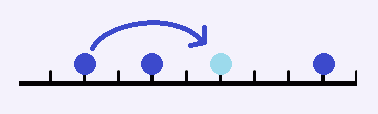# Description

跳跳棋是在一条数轴上进行的。棋子只能摆在整点上。每个点不能摆超过一个棋子。我们用跳跳棋来做一个简单的游戏：棋盘上有3颗棋子，分别在a，b，c这三个位置。我们要通过最少的跳动把他们的位置移动成x，y，z。（棋子是没有区别的）跳动的规则很简单，任意选一颗棋子，对一颗中轴棋子跳动。跳动后两颗棋子距离不变。一次只允许跳过1颗棋子。写一个程序，首先判断是否可以完成任务。如果可以，输出最少需要的跳动次数。# Input

第一行包含三个整数，表示当前棋子的位置a b c。（互不相同）第二行包含三个整数，表示目标位置x y z。（互不相同）

100% 绝对值不超过10^9

# Output

如果无解，输出一行NO。如果可以到达，第一行输出YES，第二行输出最少步数。

# Solutions

首先，根据题意容易得到一个DP。

设$f(i,j,k),i \leq j \leq k$表示三个点分别在$i,j,k$三个位置时的解。

其转移方程为

这个DP显然是过不了的，但是这个DP有些特殊性质。

在指出这个特殊性质之前，先口胡一下。

个人认为，DP本质上是基于拓扑序的图上求解，无后效性表明该DP是可拓扑的(不是很了解拓扑，如果有误，望指出)。因此，DP和图应该是可以互相转换的。

现在回到题目上来。在这个DP中，中间往两边跳的有两种，而两边往中间跳的只有一种。而且往两边跳会导致中间节点与两边距离增加，而往中间跳会导致中间节点与两边距离减小

那么，利用上面的思想，将这个DP转移成图后，这个图必定是一个森林(因为每次转移都会导致距离的增加或减小)，而且对于一个三元组$(x,y,z)$，其父亲节点是往中间跳的状态，其儿子节点是往两边跳的状态。然后答案就等价于求某棵树上两点的距离

现在的问题是如何求解树上路径距离。

最常见的方法是求$LCA$，也就是说需要有一种快速的方法求出$(x,y,z)$往中间跳$k$步的结果。

暴力跳$k$步肯定是会$T$的。设三元组$(x,y,z)$前两个的距离为$L_1=y-x$，后两个的距离为$L_2=z-y$，且假设$L1<L2$。则左边最多可以跳$\lfloor \frac{L_2-1}{L_1} \rfloor$，然后只能右边往中间跳，这样往复循环。

而且连续跳$\lfloor \frac{L_2-1}{L_1} \rfloor$次后，距离$(L_1,L_2)$会变成$(L_2 \, mod \, L_1,L_1)$，这个和欧几里得算法的递推过程一样。因此最多$log \, L$次就会结束。

于是就可以$log \, k$的时间内求出$(x,y,z)$往中间跳$k$步的结果。剩下的内容就和求普通$LCA$一样了，首先跳到同深度，然后用倍增求$LCA$。